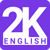# Latest Gate Aptitude Question SOLUTION: Consider the following C function: int f(int n) { static int are = 0; If (n < = 0) return 1; If (n > 3) { r = n; return f (n – 2) + 2; } return f(n – 1) + r; } What is the vLaunched an English app featuring 2000 mostly asked english words in all Competitive Exams. #2kenglish Download Now, It's Free